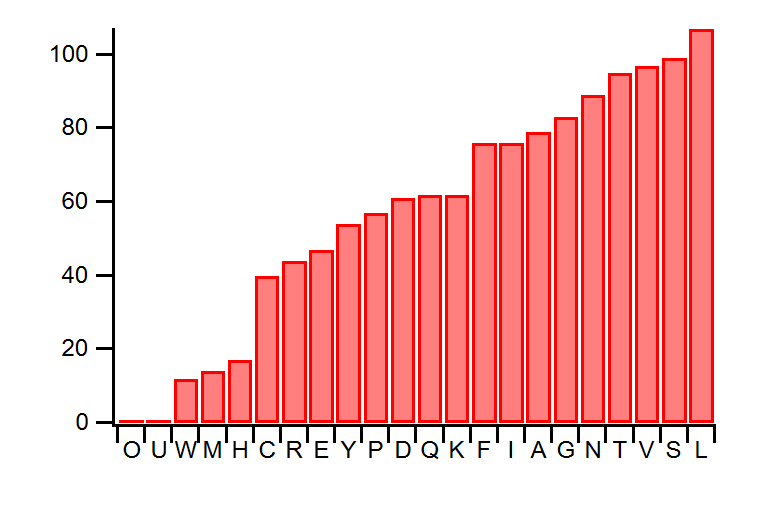# Working With Binary String Data Examples

These example functions demonstrate some uses for StringToUnsignedByteWave and WaveDataToString, two built-in functions that were introduced in Igor Pro 9.00B08 (released July 27, 2021). If you would like access to Igor Pro 9 Beta, please submit the sign up form.

`DisplayHelpTopic "Working With Binary String Data"`

### Calculating an amino acid histogram:

// Calculate a histogram of the amino acids found
// in the human SARS-CoV-2 spike protein.
Function covidSpikeAminoAcidHistogram()
String url =
URLRequest url=url
if (V_responseCode != 200)
return 0
endif
String response = S_serverResponse

// The first line in the response is a description of the
// sequence. The sequence starts after the first line feed character.
Variable sequenceStart = strsearch(response, "\n", 0)
if (sequenceStart <= 0)
print "Could not find start of sequence."
return 0
endif

String sequence = response[sequenceStart+1, inf]
// Remove any line endings in the sequence
sequence = ReplaceString("\n", sequence, "")
sequence = TrimString(sequence)

// Convert the sequence into a byte wave
WAVE/B/U seqWave = StringToUnsignedByteWave(sequence)

// Calculate the histogram with 26 bins (one for each letter).
// Start the bins at the numeric value 65, which corresponds to "A".
Histogram/C/B={char2num("A"),1,26}/DEST=seqHistWave seqWave

// Create a text wave that contains the amino acid abbreviations.
Make/O/N=26/T aminoAcidLabels
aminoAcidLabels = num2char(char2num("A") + p)

// Some letters do not correspond to an actual amino acid. So
// delete those points from both waves.
DeletePoints char2num("Z") - char2num("A"), 1, seqHistWave, aminoAcidLabels
DeletePoints char2num("X") - char2num("A"), 1, seqHistWave, aminoAcidLabels
// U is Selenocysteine. It is rare.
//  DeletePoints char2num("U") - char2num("A"), 1, seqHistWave, aminoAcidLabels
// O is pyrrolysine. It is rare.
//  DeletePoints char2num("O") - char2num("A"), 1, seqHistWave, aminoAcidLabels
DeletePoints char2num("J") - char2num("A"), 1, seqHistWave, aminoAcidLabels
DeletePoints char2num("B") - char2num("A"), 1, seqHistWave, aminoAcidLabels

// Sort the histogram wave to increasing size. Also sort
// the amino acid labels wave in the same order.
Sort seqHistWave, seqHistWave, aminoAcidLabels

// Display the histogram.
Display seqHistWave vs aminoAcidLabels
End

Here is the graph produced by executing the function:### ROT13 Encoding:

ROT13 is a simple cipher that replaces a letter with the 13th letter after it.

// Returns a ROT13 encoded string.
Function/S ROT13(String inStr)
if (!strlen(inStr) > 0) // null or empty string
return inStr
endif

Variable la = char2num("a")     // 97
Variable lz = char2num("z")     // 122
Variable bA = char2num("A")     // 65
Variable bZ = char2num("Z")     // 90

// Get the bytes
WAVE/B/U bytes = StringToUnsignedByteWave(inStr)

// encode uppercase letters
bytes = (bytes[p] >= bA && bytes[p] <= bZ) ? mod(bytes[p] - bA + 13, 26) + bA : bytes[p]

// encode lowercase letters
bytes = (bytes[p] >= lA && bytes[p] <= lZ) ? mod(bytes[p] - lA + 13, 26) + lA : bytes[p]

// convert back to a string
String outStr = WaveDataToString(bytes)

return outStr
End
print rot13("AbC+z")
NoP+m
print rot13("NoP+m")
AbC+z

### Generating Random Text:

// Returns a random printable ascii character.
// "Printable" characters include the space, tab, line feed,
// and carriage return character.
Variable c
do
c = trunc(abs(enoise(126)))
if ((c >= 32 && c <= 126) || c == 9 || c == 10 || c == 13)
break
endif
while (1)
return c
End

// Generates length bytes of random ASCII text.
Function/S RandomPrintableText(Variable length)
Make/FREE/B/U/N=(length) chars
String theText = WaveDataToString(chars)
return theText
End
print RandomPrintableText(10)
YI2XcCsPR9
print RandomPrintableText(10)
18'Gf[C\O%

### Remove Non-printable Characters From a String

An example of how to remove non-printable characters from a string is given in a separate code snippet.ForumSupportGallery